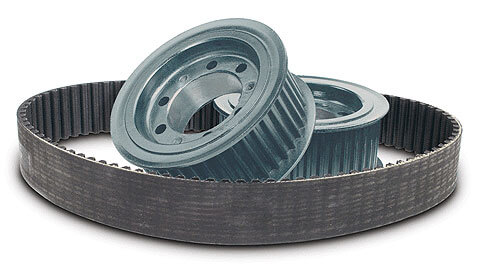# Timing Belts and Pulleys: Introduction to Speed Ratio

Synchronous drive systems operate by the positive engagement of meshing teeth between the timing pulley and timing belt.  These drives are denoted as such because they maintain constant system synchronicity with no relative motion losses in the drive.   In this way, these drive systems can be primarily characterized by a consistent speed ratio.

The speed ratio is perhaps the most basic yet most important concept to grasp at the very beginning of the belt drive selection process.  The speed ratio essentially gets the operational speed of the equipment and defines the geometric shape and size envelope of the drive system and, as such, this parameter is an integral and essential part of the drive selection calculation.

Despite its sizing importance, the concept of the speed ratio is very simple to grasp, yet is extremely useful for the sizing of pulley drive systems.  The speed ratio is defined as the ratio of the large to small pulley size and can be calculated simply by dividing the number of teeth in the large pulley by the number of teeth in the small pulley.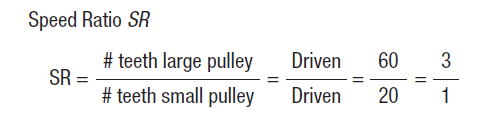With the calculation formulated in this way, the speed ratio will always be a value greater than 1.0, so the drive system designer engineer can easily identify a mistake if a ratio less than 1.0 is somehow mistakenly calculated.  However, the engineer must recognize the speed of the drive system can either be sped up (smaller pulley as the driver pulley) or slowed down (larger pulley is the driver pulley).

Certainly, it’s not difficult to see how the speed ratio characterizes the geometry of the drive system because it is literally a ratio of the pulley sizes. Typically, timing pulley systems do not use speed ratios larger than 5:1. However, with the right system layout higher rations can be achieved.

Linear/ Rotational Speed Relation

It is largely up to the system designer to determine the speed ratio of the system as it is dictated by the needs of the drive system, but sometimes there are certain design requirements that provide just enough information to calculate the speed ratio.

The speed ratio can be determined considering the relative pulley speeds due to the fact that angular velocity of the driving systems is proportional related such that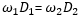.  Whereand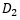are the pulley sizes, and  and  are the angular velocities of these pulleys, respectively.  This expression exists because of the fact that in synchronous drive systems, the linear velocity of the belt linking the two drives must always be a constant value.  In this way, the angular velocities of the two pulley belt drives can be used to determine the speed ratio by the expression: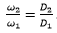.  It’s not hard to see that in this expression the speed ratio is simply the ratio of the two pulley diameters –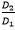.

A common design scenario is one where the belt drive systems is either driven by belt speed requirements of the system or limited by the size envelope of pulleys.  If a linear velocity requirement is imposed on a conceptual synchronous belt drive system, then the designer can leverage these calculations to help calculate the speed ratio.  All that is needed to accomplish this and fully characterize the speed ratio along with the rough geometry of the drive is the size of one of the pulleys in the system.

In this way, from a very limited set of design information, the system designer can quickly determine the key geometric parameter of the drive system and gain a solid head start on the sizing calculations.

Use in Drive System Calculations

The speed ratio of the system is important to calculate for a number of reasons, one of which being that it is used to determine the Speed Ratio Correction Factor (Kr) which is used to formulate the Overload Correction factor (Ks).  This factor is a critical design parameter that is necessary for determining the system Design Power (Pd) that the belt and pulley must be capable of transmitting.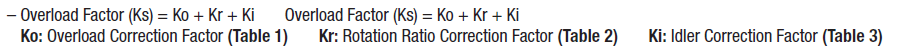Typically, the speed ratio is a predetermined system design factor.  From here, the number of teeth on the small pulley and large pulley can be specified in order to fit the speed ratio.  However, there are limits to the minimum number of teeth that can be specified in the smallest drive factor; this factor is specified in the MISUMI 1st Edition Configurable Timing Pulleys and Timing Belts.

Conclusion

Once the system design power Pd has been determined, either by design or by calculation, the rest of the belt drive selection process pretty much flows downhill from here with a few other design parameter calculations that are necessary in order to design a synchronous drive system.  The MISUMI 1st Edition Configurable Timing Pulleys and Timing Belts contains all of the details and calculations that are required to completely size each drive system component for a wide range of different timing belt drives and pulley applications.

## Under Pressure: Pneumatic Circuits

Do you hear that air? The click, the ...